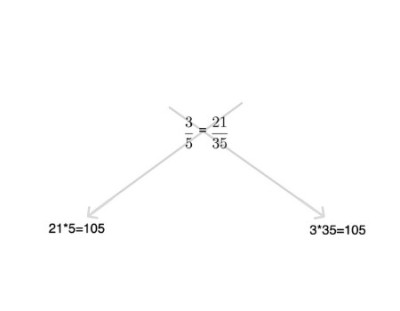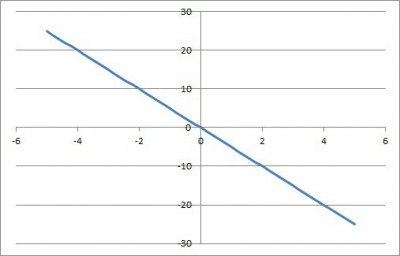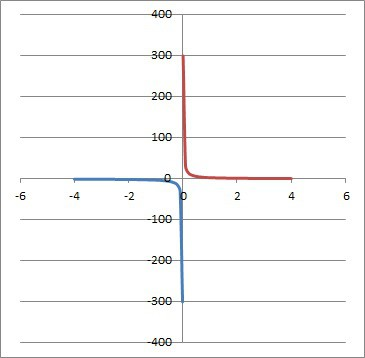# How To Solve Proportions (3 Things To Know)

Proportions come up often in algebra, probability, calculus, and other subjects outside of mathematics.  It helps to be comfortable with solving proportions – luckily, there is an easy way to get a simpler equation from a proportion.

So, how do you solve proportions?  To solve a proportion, first use cross multiplication to get an equation without fractions. Next, simplify any parentheses and combine like terms. Then, solve the equation for the variable.  Finally, test the value of the variable in the original proportion to verify the solution.

Although some proportions have a single solution, there are others that have two or more solutions, and some that have no solution at all.

In this article, we’ll look at how to solve proportions with several examples.  We’ll also examine where cross multiplication comes from and why it works to solve a proportion.

Let’s get started.

## How To Solve Proportions

Remember that a proportion is an equation that sets two ratios (fractions) equal to each other.  For example, the equation 4/6 = 2/3 is a proportion.

In a proportion, both fractions have the same value (possibly in a different form, as we saw in the example above).

Proportions can be used to solve similar triangles, among other things.

Some proportions have one or more variables in them.  To solve a proportion with a variable, we use cross multiplication.

### What Is Cross Multiplication?

Cross multiplication takes a proportion and turns it into an equation with no fractions.  The new equation is equivalent to the original proportion (and it has the same solutions if there are variables).

Given a proportion a/b = x/y, cross multiplication gives us the equivalent equation:

• ay = bxCross multiplication of a proportion allows us to convert the equation to another one without fractions.

Here is a proof of why cross multiplication works:

• a/b = x/y  [this is the original proportion]
• b(a/b) = b(x/y)  [multiply by b on both sides]
• a = bx/y  [the factor of b cancels on the left side]
• y(a) = y(bx/y)  [multiply by y on both sides]
• ay = bx  [the factor of y cancels on the right side]

Cross multiplication helps us to solve proportions by giving us an equation without fractions.  The resulting equation is easier to solve.

#### Example 1: Using Cross Multiplication To Solve A Proportion

Let’s say we want to solve the proportion 4/x = 2/3.  Using cross multiplication, we get:

• 4*3 = x*2
• 12 = 2x
• 6 = x

We can test this solution in the original proportion to verify that it is correct:

• 4/x = 2/3
• 4/6 = 2/3
• 2/3 = 2/3

So, the solution to the proportion4/x = 2/3 is x = 6.

#### Example 2: Using Cross Multiplication To Solve A Proportion

Let’s say we want to solve the proportion 3/(x – 1) = 2/5.  Using cross multiplication, we get:

• 3*5 = (x – 1)*2
• 15 = 2x – 2
• 17 = 2x
• 17/2 = x
• 8.5 = x

We can test this solution in the original proportion to verify that it is correct:

• 3/(x – 1) = 2/5
• 3/(8.5 – 1) = 2/5
• 3/(7.5) = 2/5
• 30/75 = 2/5
• 2/5 = 2/5

#### Example 3: Using Cross Multiplication To Solve A Proportion

Let’s say we want to solve the proportion 7/(2x – 3) = 5/x  Using cross multiplication, we get:

• 7*x = (2x – 3)*5
• 7x = 10x – 15
• -3x = -15
• x = 5

We can test this solution in the original proportion to verify that it is correct:

• 7/(2x – 3) = 5/x
• 7/(2(5) – 3) = 5/5
• 7/(10 – 3) = 1
• 7 / 7 = 1
• 1 = 1

#### Example 4: Using Cross Multiplication To Solve A Proportion

Let’s say we want to solve the proportion 2/(x – 2) = (x – 3)/x  Using cross multiplication, we get:

• 2*x = (x – 2)(x – 3)
• 2x = x2 – 5x + 6
• 0 = x2 – 7x + 6
• 0 = (x – 1)(x – 6)
• x = 1 or x = 6

We can test both of these solutions in the original proportion to verify that that they are correct.

For x = 1:

• 2/(x – 2) = (x – 3)/x
• 2/(1 – 2) = (1 – 3)/1
• 2 / -1 = -2 / 1
• -2 = -2

For x = 6:

• 2/(x – 2) = (x – 3)/x
• 2/(6 – 2) = (6 – 3)/6
• 2 / 4 = 3 / 6
• 1/2 = 1/2

#### Example 5: Using Cross Multiplication To Solve A Proportion

Let’s say we want to solve the proportion (x – 1)/(x – 2) = (x – 4)/(x – 8)  Using cross multiplication, we get:

• (x – 1)(x – 8) = (x – 2)(x – 4)
• x2 – 9x + 8 = x2 – 6x + 8
• -9x = -6x
• -3x = 0
• x = 0

We can test this solution in the original proportion to verify that that it is correct:

• (x – 1)/(x – 2) = (x – 4)/(x – 8)
• (0 – 1)/(0 – 2) = (0 – 4)/(0 – 8)
• -1 / -2 = -4 / -8
• 1/2 = 1/2

#### Example 6: Using Cross Multiplication To Solve A Proportion

Let’s say we want to solve the proportion (x – 2)/(x – 3) = (x – 4)/(x – 7)  Using cross multiplication, we get:

• (x – 2)(x – 7) = (x – 3)(x – 4)
• x2 – 9x + 14 = x2 – 7x + 12
• -9x + 14 = -7x + 12
• 14 = 2x + 12
• 2 = 2x
• 1 = x

We can test this solution in the original proportion to verify that that it is correct:

• (x – 2)/(x – 3) = (x – 4)/(x – 7)
• (1 – 2)/(1 – 3) = (1 – 4)/(1 – 7)
• -1 / -2 = -3 / -6
• 1/2 = 1/2

#### Example 7: Using Cross Multiplication To Solve A Proportion

Let’s say we want to solve the proportion 4/x2 = x/2.  Using cross multiplication, we get:

• 4*2 = x2*x
• 8 = x3
• 2 = x

We can test this solution in the original proportion to verify that that it is correct:

• 4/x2 = x/2
• 4/22 = 2/2
• 4/4 = 1
• 1 = 1

#### Example 8: Using Cross Multiplication To Solve A Proportion

Let’s say we want to solve the proportion 2/(x2 + x – 4) = x/2.  Using cross multiplication, we get:

• 2*2 = (x2 + x – 4)*x
• 4 = x3 + x2 – 4x
• 0 = x3 + x2 – 4x – 4
• 0 = (x3 + x2) – 4x – 4
• 0 = x2(x + 1) – 4(x + 1)
• 0 = (x2 – 4)(x + 1)
• 0 = (x – 2)(x + 2)(x + 1)
• x = 2, -2, -2

We can test these solutions in the original proportion to verify that that they are correct:

For x = 2:

• 2/(x2 + x – 4) = x/2
• 2/(22 + 2 – 4) = 2/2
• 2/(4 + 2 – 4) = 1
• 2/(2) = 1
• 1 = 1

For x = -2:

• 2/(x2 + x – 4) = x/2
• 2/((-2)2 + -2 – 4) = -2/2
• 2/(4 + -2 – 4) = -1
• 2/(-2) = -1
• -1 = -1

For x = -1:

• 2/(x2 + x – 4) = x/2
• 2/((-1)2 + -1 – 4) = -1/2
• 2/(1 + -1 – 4) = -1/2
• 2/(- 4) = -1/2
• -1/2 = -1/2

#### Example 9: Using Cross Multiplication To Solve A Proportion

Let’s say we want to solve the proportion 1/x = 2/x.  Using cross multiplication, we get:

• 1*x = x*2
• x = 2x
• 0 = x

When we test this solution in the original proportion, we get zero denominators.  So, there is no solution for this proportion.

#### Example 10: Using Cross Multiplication To Solve A Proportion

Let’s say we want to solve the proportion (x – 2)/(x – 3) = (x – 4)/(x – 5).  Using cross multiplication, we get:

• (x – 2)(x – 5) = (x – 3)(x – 4)
• x2 – 7x + 10 = x2 – 7x + 12
• 10 = 12

Since this equation is never true, there is no solution to this proportion.

## What Is A Direct Proportion?

Two variables x and y are a direct proportion if y = kx for some constant k (k cannot be zero).

Visually, this means that the graph will be a non-horizontal, non-vertical line that passes through the origin (0, 0).The line y = -5x goes through the origin (0, 0). It is a direct proportion between x and y. In this case, the constant is k = -5.

We can solve for the constant k as follows:

• y = kx
• y/x = k
• y/x = k/1

So, a proportion that relates x and y can be simplified to the equation we saw earlier, y = kx.

## What Is An Inverse Proportion?

Two variables x and y are an inverse proportion if y = k/x for some constant k (k cannot be zero).

Visually, this means that the graph will be a curve with vertical asymptote at x = 0 and horizontal asymptote at y = 0.The curve xy = 3 (or y = 3/x) has a vertical asymptote at x = 0 and a vertical asymptote at y = 0. It is an inverse proportion between x and y. In this case, the constant is k = 3.

We can solve for the constant k as follows:

• y = k/x
• yx = k

So, the product of the variables x and y is always a constant, k.  We can also write the proportion y/1 = k/x as an equivalent form of the inverse variation equation we saw earlier.

## Conclusion

Now you know how to solve proportions, whether the resulting equations are linear, quadratic, cubic, or otherwise.  You also know about direct and inverse proportions.